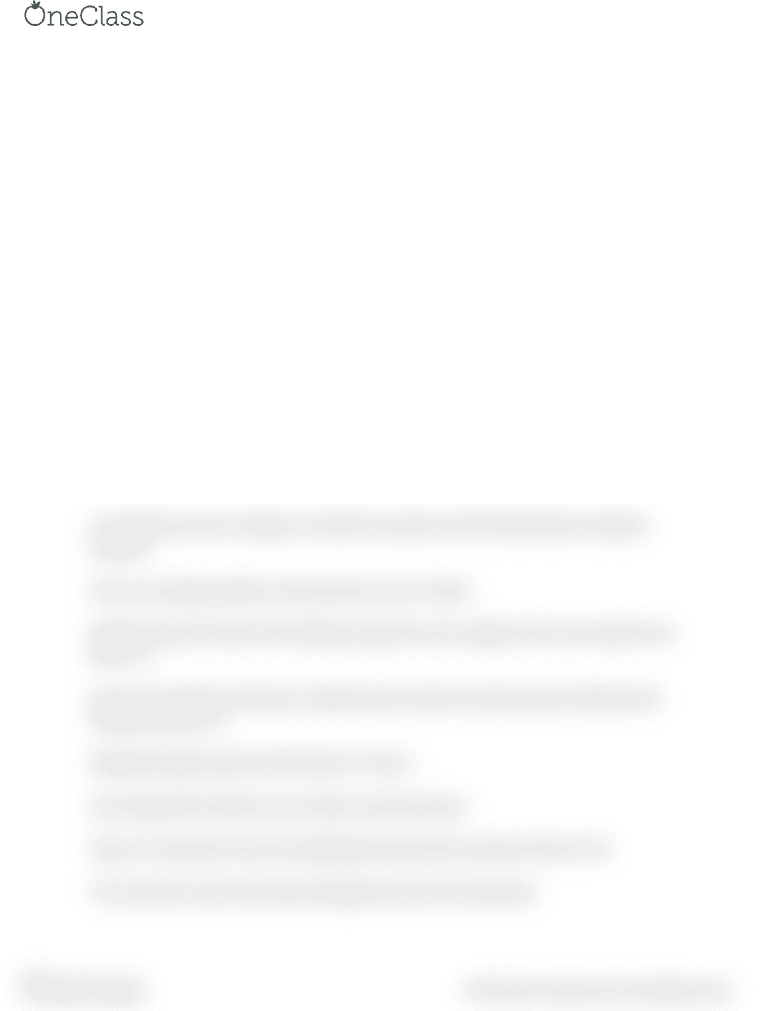# CHEM1006 Lecture Notes - Lecture 3: Conversion Of Units, Dimensional Analysis

21 views2 pages
School
Department
CourseLecture 3
Chemistry, along with other sciences and engineering, makes use of many different units.
Some of the common ones include mass; length; and energy.
Since there are so many different units that can be used, it is necessary to be able to convert
between the various units.
In mathematics, specifically algebra, a conversion factor is used to convert a measured quantity
to a different unit of measure without changing the relative amount.
To accomplish this, a ratio is established that equals one.
In the ratio, the conversion factor is a multiplier that, when applied to the original unit,
converts the original unit into a new unit, by multiplication with the ratio.
Identify conversion factors that will help you get from your original units to your desired unit.
Set up your equation so that your undesired units cancel out to give you your desired units.
A unit will cancel out if it appears in both the numerator and the denominator during the
equation.
Here is an example problem: How many hours are in 3 days?
Identify conversion factors that will help you get from your original units to your desired unit:
\$frac \$. 3.
Set up your equation so that your undesired units cancel out to give you your desired units:
\$3:days cdot frac \$. 4.
Multiply through to get your final answer: 72 hours.
Don't forget that if need be, you can flip a conversion factor.
Days are converted to hours by multiplying the days by the conversion factor of 24.
The conversion can be reversed by dividing the hours by 24 to get days.
find more resources at oneclass.com
find more resources at oneclass.com
Unlock document

This preview shows half of the first page of the document.
Unlock all 2 pages and 3 million more documents.

Already have an account? Log in

# Get access

Grade+
\$10 USD/m
Billed \$120 USD annually
Homework Help
Class Notes
Textbook Notes
40 Verified Answers
Study Guides
1 Booster Class
Class+
\$8 USD/m
Billed \$96 USD annually
Homework Help
Class Notes
Textbook Notes
30 Verified Answers
Study Guides
1 Booster Class